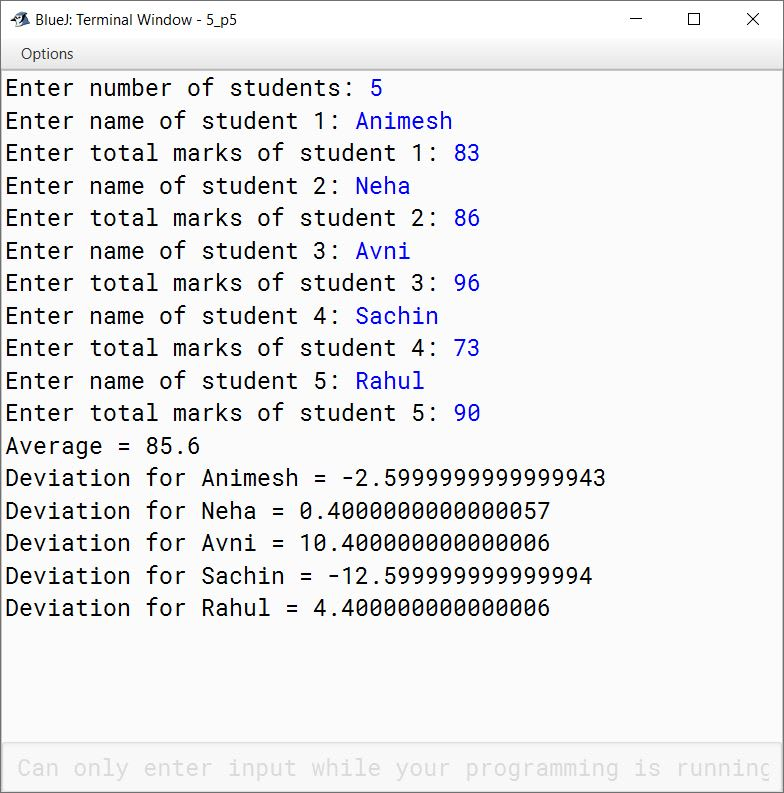# Solved 2018 Question Paper ICSE Class 10 Computer Applications

## Section A

#### Question 1

(a) Define abstraction.

Abstraction refers to the act of representing essential features without including the background details or explanation.

(b) Differentiate between searching and sorting.

SearchingSorting
Searching means to search for a term or value in an array.Sorting means to arrange the elements of the array in ascending or descending order.
Linear search and Binary search are examples of search techniques.Bubble sort and Selection sort are examples of sorting techniques.

(c) Write a difference between the functions isUpperCase( ) and toUpperCase( ).

isUpperCase( )toUpperCase( )
isUpperCase( ) function checks if a given character is in uppercase or not.toUpperCase( ) function converts a given character to uppercase.
Its return type is boolean.Its return type is char.

(d) How are private members of a class different from public members?

private members of a class can only be accessed by other member methods of the same class whereas public members of a class can be accessed by methods of other classes as well.

(e) Classify the following as primitive or non-primitive datatypes:

1. char
2. arrays
3. int
4. classes

1. Primitive
2. Non-Primitive
3. Primitive
4. Non-Primitive

#### Question 2

(a) (i) int res = 'A';
What is the value of res?

Value of res is 65 which is the ASCII code of A. As res is an int variable and we are trying to assign it the character A so through implicit type conversion the ASCII code of A is assigned to res.

(ii) Name the package that contains wrapper classes.

java.lang

(b) State the difference between while and do while loop.

whiledo-while
It is an entry-controlled loop.It is an exit-controlled loop.
It is helpful in situations where numbers of iterations are not known.It is suitable when we need to display a menu to the user.

(c) System.out.print("BEST ");
System.out.println("OF LUCK");

Choose the correct option for the output of the above statements

1. BEST OF LUCK
2. BEST
OF LUCK

Option 1 — BEST OF LUCK is the correct option.
System.out.print does not print a newline at the end of its output so the println statement begins printing on the same line. So the output is BEST OF LUCK printed on a single line.

(d) Write the prototype of a function check which takes an integer as an argument and returns a character.

char check(int a)

(e) Write the return data type of the following function.

1. endsWith()
2. log()

1. boolean
2. double

#### Question 3

(a) Write a Java expression for the following:

$\bold{\dfrac{\sqrt{3x+x^2}}{a + b}}$

Math.sqrt(3 * x + x * x) / (a + b)

(b) What is the value of y after evaluating the expression given below?

y+= ++y + y-- + --y; when int y=8

y+= ++y + y-- + --y
⇒ y = y + (++y + y-- + --y)
⇒ y = 8 + (9 + 9 + 7)
⇒ y = 8 + 25
⇒ y = 33

(c) Give the output of the following:

1. Math.floor (-4.7)
2. Math.ceil(3.4) + Math.pow(2, 3)

1. -5.0
2. 12.0

(d) Write two characteristics of a constructor.

Two characteristics of a constructor are:

1. A constructor has the same name as its class.
2. A constructor does not have a return type.

(e) Write the output for the following:

System.out.println("Incredible"+"\n"+"world");

Output of the above code is:

Incredible
world


\n is the escape sequence for newline so Incredible and world are printed on two different lines.

(f) Convert the following if else if construct into switch case

if( var==1)
System.out.println("good");
else if(var==2)
System.out.println("better");
else if(var==3)
System.out.println("best");
else
System.out.println("invalid");

switch (var) {
case 1:
System.out.println("good");
break;

case 2:
System.out.println("better");
break;

case 3:
System.out.println("best");
break;

default:
System.out.println("invalid");
}

(g) Give the output of the following string functions:

1. "ACHIEVEMENT".replace('E', 'A')
2. "DEDICATE".compareTo("DEVOTE")

1. ACHIAVAMANT
2. -18
##### Explanation
1. "ACHIEVEMENT".replace('E', 'A') will replace all the E's in ACHIEVEMENT with A's.
2. The first letters that are different in DEDICATE and DEVOTE are D and V respectively. So the output will be:
ASCII code of D - ASCII code of V
⇒ 68 - 86
⇒ -18

(h) Consider the following String array and give the output

String arr[]= {"DELHI", "CHENNAI", "MUMBAI", "LUCKNOW", "JAIPUR"};
System.out.println(arr.length() > arr.length());
System.out.print(arr.substring(0,3));

Output of the above code is:

false
JAI

##### Explanation
1. arr is DELHI and arr is LUCKNOW. Length of DELHI is 5 and LUCKNOW is 7. As 5 > 7 is false so the output is false.
2. arr is JAIPUR. arr.substring(0,3) extracts the characters from index 0 till index 2 of JAIPUR so JAI is the output.

(i) Rewrite the following using ternary operator:

if (bill > 10000 )
discount = bill * 10.0/100;
else
discount = bill * 5.0/100;

discount = bill > 10000 ? bill * 10.0/100 : bill * 5.0/100;

(j) Give the output of the following program segment and also mention how many times the loop is executed:

int i;
for ( i = 5 ; i > 10; i ++ )
System.out.println( i );
System.out.println( i * 4 );

Output of the above code is:

20


Loop executes 0 times.

##### Explanation

In the loop, i is initialized to 5 but the condition of the loop is i > 10. As 5 is less than 10 so the condition of the loop is false and it is not executed. There are no curly braces after the loop which means that the statement System.out.println( i ); is inside the loop and the statement System.out.println( i * 4 ); is outside the loop. Loop is not executed so System.out.println( i ); is not executed but System.out.println( i * 4 ); being outside the loop is executed and prints the output as 20 (i * 4 ⇒ 5 * 4 ⇒ 20).

## Section B

#### Question 4

Design a class RailwayTicket with following description:

Instance variables/data members:
String name — To store the name of the customer
String coach — To store the type of coach customer wants to travel
long mobno — To store customer’s mobile number
int amt — To store basic amount of ticket
int totalamt — To store the amount to be paid after updating the original amount

Member methods:
void accept() — To take input for name, coach, mobile number and amount.
void update() — To update the amount as per the coach selected (extra amount to be added in the amount as follows)

Type of CoachesAmount
First_AC700
Second_AC500
Third_AC250
sleeperNone

void display() — To display all details of a customer such as name, coach, total amount and mobile number.

Write a main method to create an object of the class and call the above member methods.

import java.util.Scanner;

public class RailwayTicket
{
private String name;
private String coach;
private long mobno;
private int amt;
private int totalamt;

private void accept() {
Scanner in = new Scanner(System.in);
System.out.print("Enter name: ");
name = in.nextLine();
System.out.print("Enter coach: ");
coach = in.nextLine();
System.out.print("Enter mobile no: ");
mobno = in.nextLong();
System.out.print("Enter amount: ");
amt = in.nextInt();
}

private void update() {
if(coach.equalsIgnoreCase("First_AC"))
totalamt = amt + 700;
else if(coach.equalsIgnoreCase("Second_AC"))
totalamt = amt + 500;
else if(coach.equalsIgnoreCase("Third_AC"))
totalamt = amt + 250;
else if(coach.equalsIgnoreCase("Sleeper"))
totalamt = amt;
}

private void display() {
System.out.println("Name: " + name);
System.out.println("Coach: " + coach);
System.out.println("Total Amount: " + totalamt);
System.out.println("Mobile number: " + mobno);
}

public static void main(String args[]) {
RailwayTicket obj = new RailwayTicket();
obj.accept();
obj.update();
obj.display();
}
}
##### Output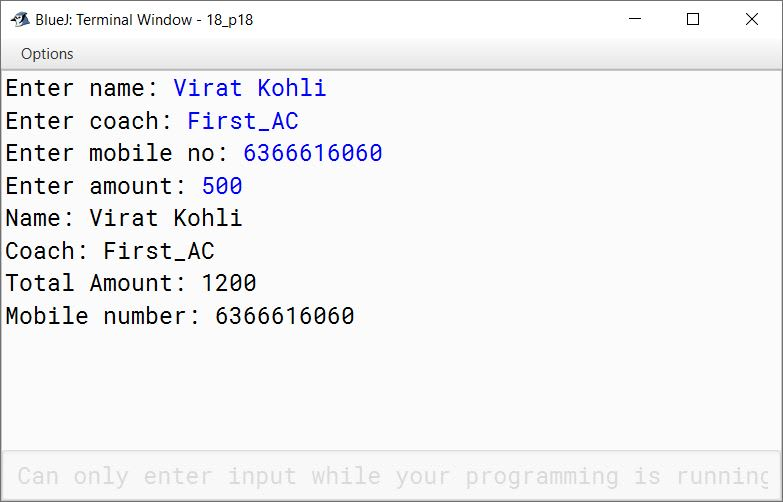#### Question 5

Write a program to input a number and check and print whether it is a Pronic number or not. (Pronic number is the number which is the product of two consecutive integers)

Examples:
12 = 3 x 4
20 = 4 x 5
42 = 6 x 7

import java.util.Scanner;

public class KboatPronicNumber
{
public static void main(String args[]) {
Scanner in = new Scanner(System.in);
System.out.print("Enter the number to check: ");
int num = in.nextInt();

boolean isPronic = false;

for (int i = 1; i <= num - 1; i++) {
if (i * (i + 1) == num) {
isPronic = true;
break;
}
}

if (isPronic)
System.out.println(num + " is a pronic number");
else
System.out.println(num + " is not a pronic number");
}
}
##### Output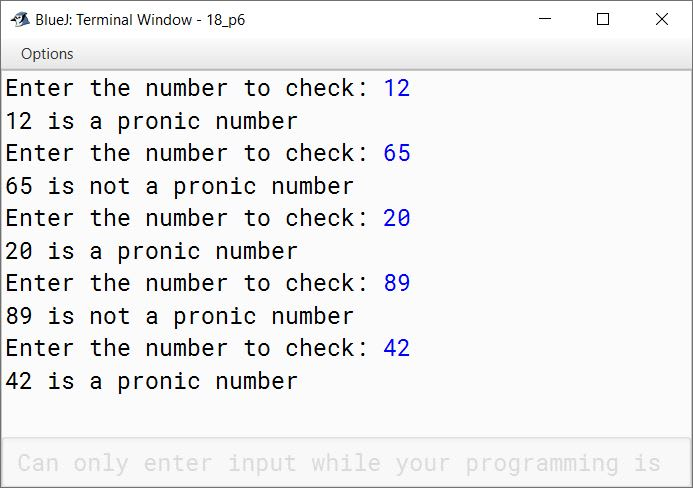#### Question 6

Write a program in Java to accept a string in lower case and change the first letter of every word to upper case. Display the new string.

Sample input: we are in cyber world
Sample output: We Are In Cyber World

import java.util.Scanner;

public class KboatString
{
public static void main(String args[]) {
Scanner in = new Scanner(System.in);
System.out.println("Enter a sentence:");
String str = in.nextLine();
String word = "";

for (int i = 0; i < str.length(); i++) {
if (i == 0 || str.charAt(i - 1) == ' ') {
word += Character.toUpperCase(str.charAt(i));
}
else {
word += str.charAt(i);
}
}

System.out.println(word);
}
}
##### Output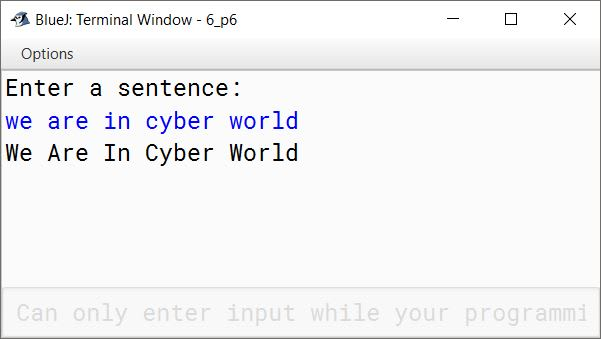#### Question 7

Design a class to overload a function volume() as follows:

1. double volume (double R) – with radius (R) as an argument, returns the volume of sphere using the formula.
V = 4/3 x 22/7 x R3

2. double volume (double H, double R) – with height(H) and radius(R) as the arguments, returns the volume of a cylinder using the formula.
V = 22/7 x R2 x H

3. double volume (double L, double B, double H) – with length(L), breadth(B) and Height(H) as the arguments, returns the volume of a cuboid using the formula.
V = L x B x H

public class KboatVolume
{
double volume(double r) {
return (4 / 3.0) * (22 / 7.0) * r * r * r;
}

double volume(double h, double r) {
return (22 / 7.0) * r * r * h;
}

double volume(double l, double b, double h) {
return l * b * h;
}

public static void main(String args[]) {
KboatVolume obj = new KboatVolume();
System.out.println("Sphere Volume = " +
obj.volume(6));
System.out.println("Cylinder Volume = " +
obj.volume(5, 3.5));
System.out.println("Cuboid Volume = " +
obj.volume(7.5, 3.5, 2));
}
}
##### Output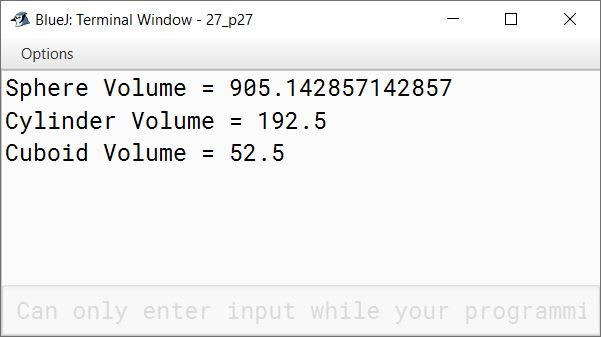#### Question 8

Write a menu driven program to display the pattern as per user’s choice.

Pattern 1

ABCDE
ABCD
ABC
AB
A

Pattern 2

B
LL
UUU
EEEE

For an incorrect option, an appropriate error message should be displayed.

import java.util.Scanner;

{
public static void main(String args[]) {
Scanner in = new Scanner(System.in);
System.out.println("Enter 1 for pattern 1");
System.out.println("Enter 2 for Pattern 2");
int choice = in.nextInt();
switch (choice) {
case 1:
for (int i = 69; i >= 65; i--) {
for (int j = 65; j <= i; j++) {
System.out.print((char)j);
}
System.out.println();
}
break;

case 2:
String word = "BLUE";
int len = word.length();
for(int i = 0; i < len; i++) {
for(int j = 0; j <= i; j++) {
System.out.print(word.charAt(i));
}
System.out.println();
}
break;

default:
System.out.println("Incorrect choice");
break;

}
}
}
##### Output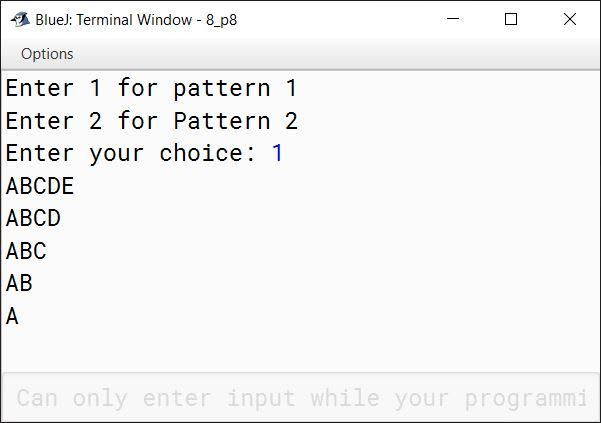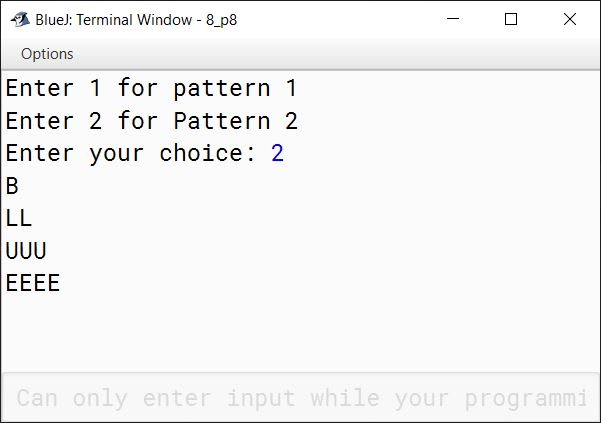#### Question 9

Write a program to accept name and total marks of N number of students in two single subscript array name[] and totalmarks[].

Calculate and print:

1. The average of the total marks obtained by N number of students.
[average = (sum of total marks of all the students)/N]
2. Deviation of each student’s total marks with the average.
[deviation = total marks of a student – average]

import java.util.Scanner;

public class KboatSDAMarks
{
public static void main(String args[]) {
Scanner in = new Scanner(System.in);
System.out.print("Enter number of students: ");
int n = in.nextInt();

String name[] = new String[n];
int totalmarks[] = new int[n];
int grandTotal = 0;

for (int i = 0; i < n; i++) {
in.nextLine();
System.out.print("Enter name of student " + (i+1) + ": ");
name[i] = in.nextLine();
System.out.print("Enter total marks of student " + (i+1) + ": ");
totalmarks[i] = in.nextInt();
grandTotal += totalmarks[i];
}

double avg = grandTotal / (double)n;
System.out.println("Average = " + avg);

for (int i = 0; i < n; i++) {
System.out.println("Deviation for " + name[i] + " = "
+ (totalmarks[i] - avg));
}
}
}
##### Output